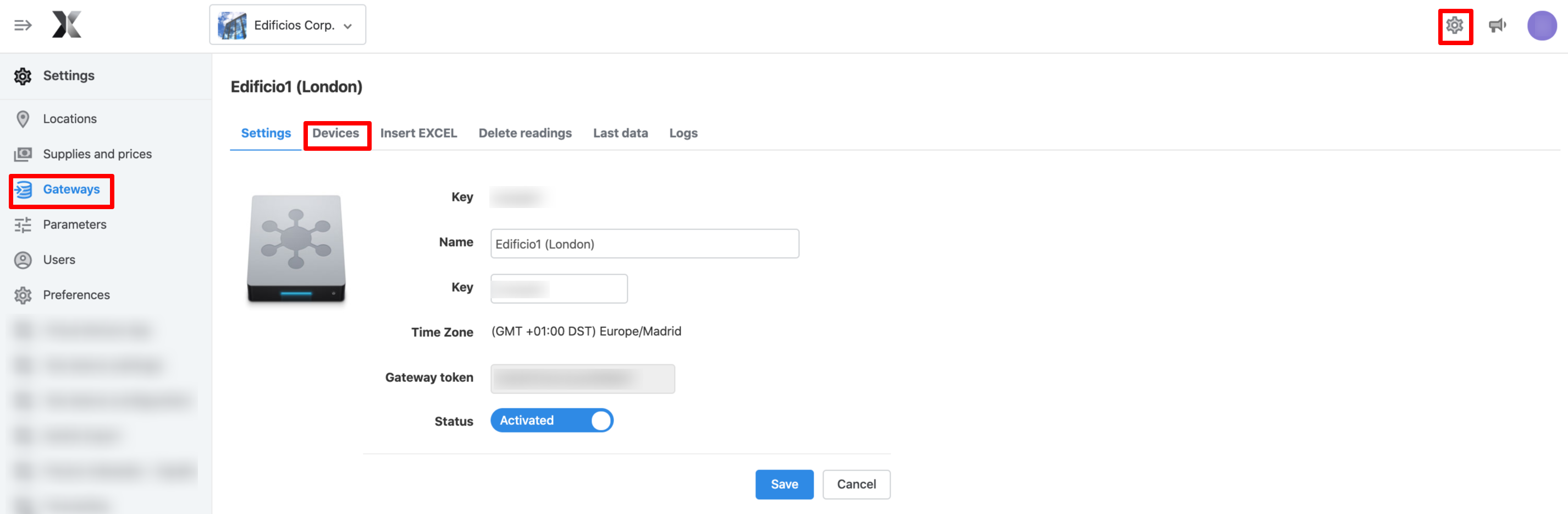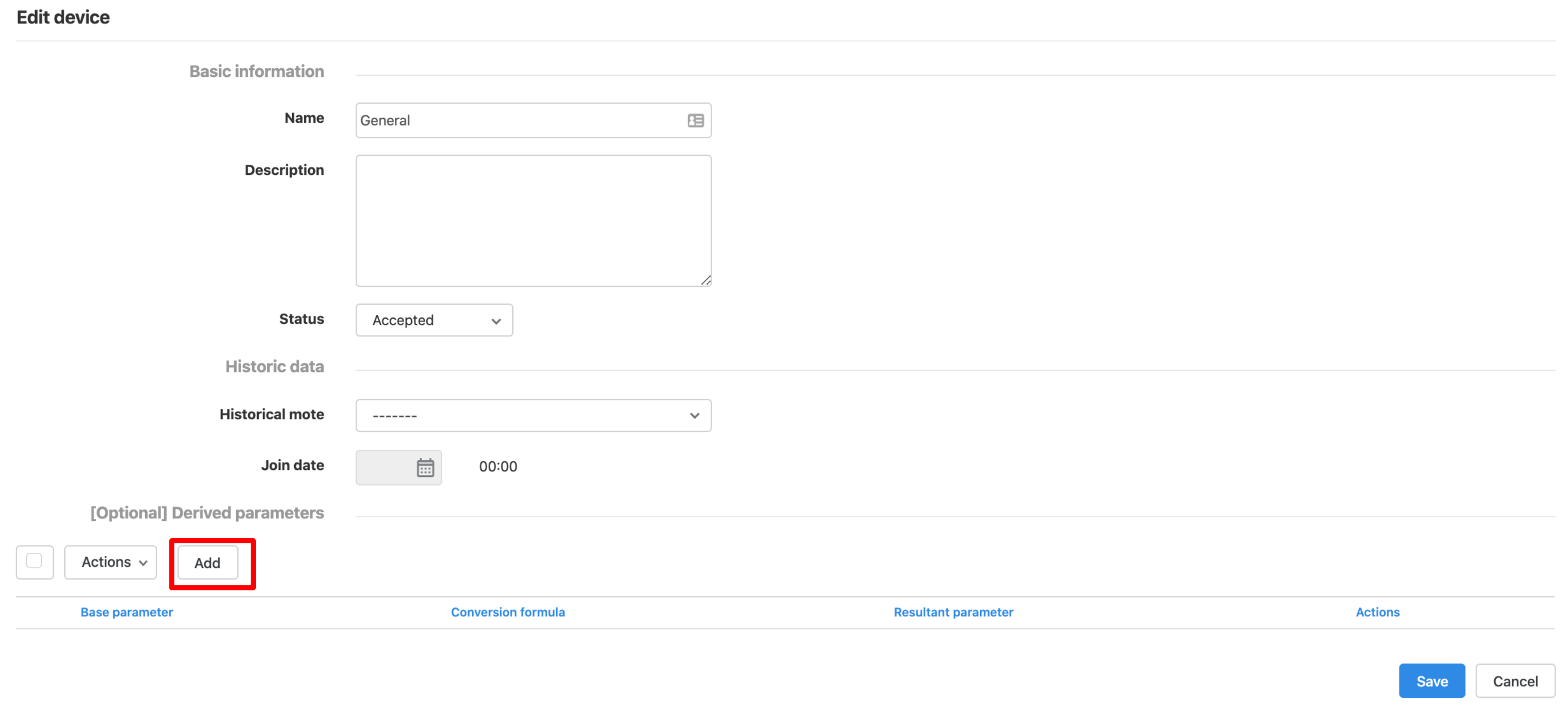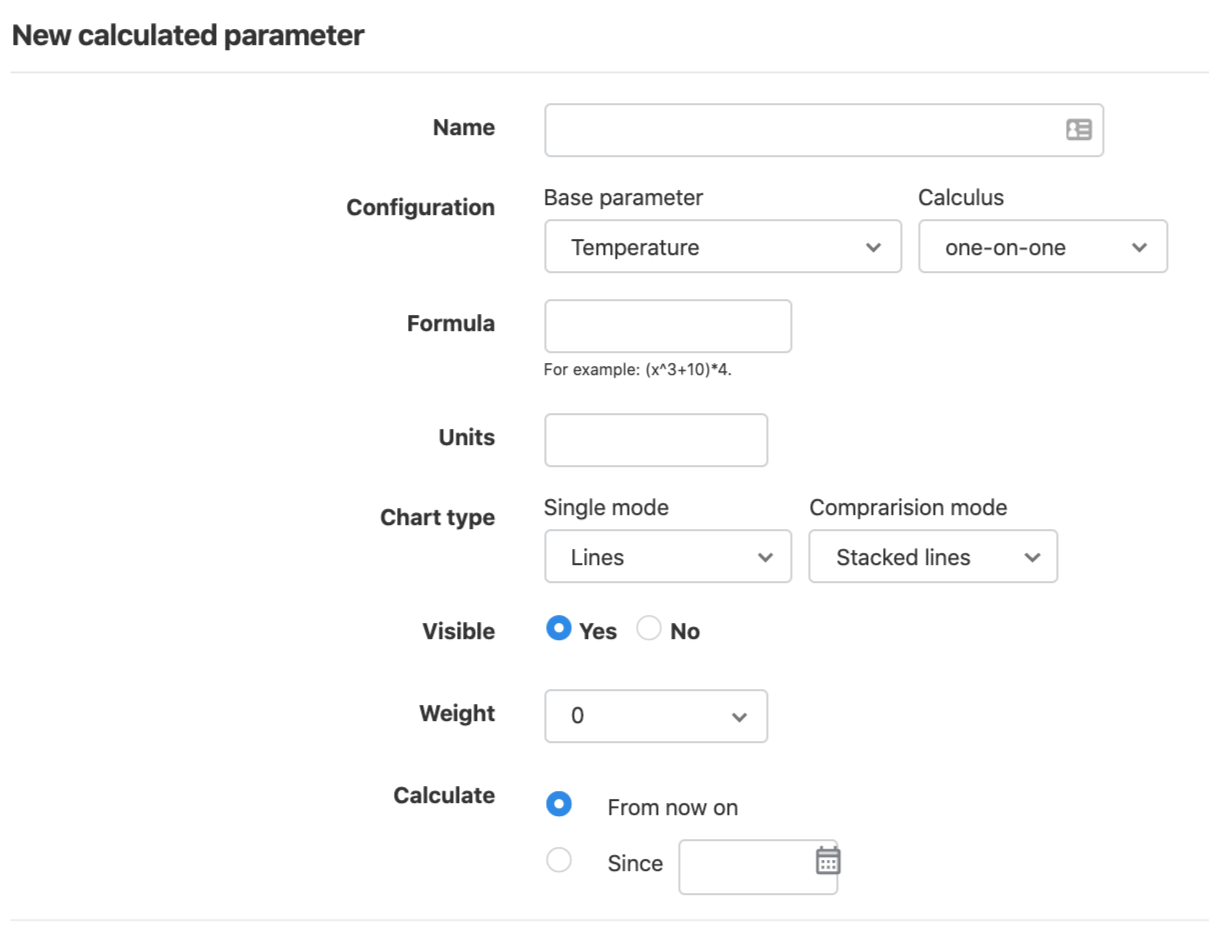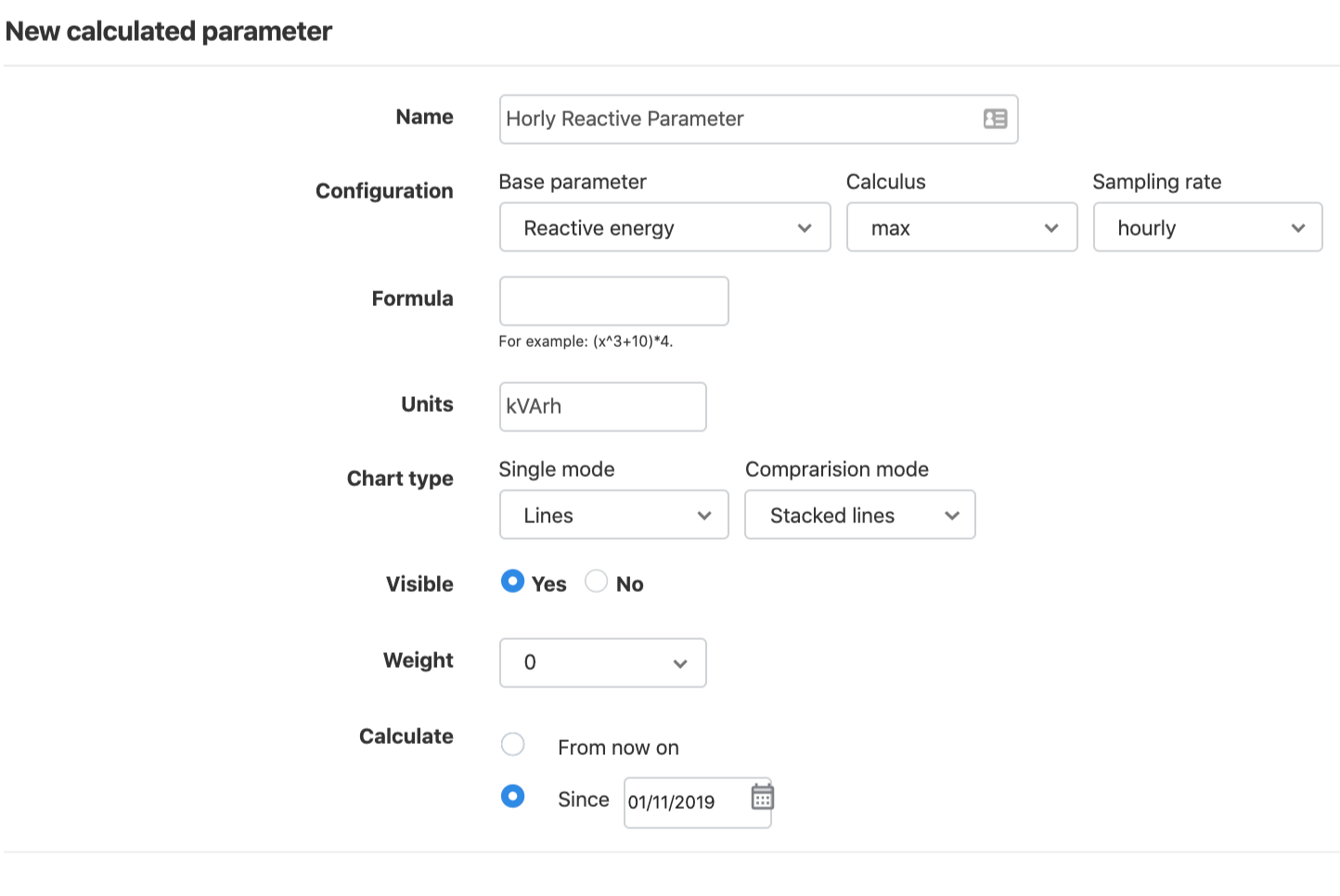# Derived and Calculated Parameters

## Introduction

In the energy management platform you can visualise parameters with values created from other inserted parameter. Below are the options that you have depending on your needs:

• If you do not want a new parameter but to visualise consumption data normalised by a value, you might be interested in ratios. Example: You measure lighting electric consumption divided by a fixed number of lamps (kWh/lamp)
• The simplest way to have a parameter obtained by another is creating a Derived parameter inside a device. Example: You measure and send pulses (502) to the platform and you want to transform them into Gas energy in kWh (420).
• If you want a derived parameter, but the new parameter is not one of the default parameters in the list, you need a Custom Parameter. Example: You measure produced units (131) and you want to see the produced kg, knowing that each unit is X kg.
• If you want a parameter that is a simple calculation between more than one parameter in the same location, you can use Groups. Example: You measure electric consumtion from the first floor and electric consumption from the second floor and you want to see the total consumption.
• If you want a parameter that results from any calculation with one or more parameters and these are not in the same location, you need Virtual Devices app. Example: You measure electric consumption and gas energy from different buildings and you want to sum them (and also divide them by some number or do some other calculation with another parameter ¿Why not?)

Below, the derived parameters are explained and also calculated parameters, which are not in use any more.

## Derived parameters

### Create Derived parameters

Following this manual, you will be able to convert a parameter into another one, simply doing a bypass or converting it through conversion factors.

This is useful when the gateway does not send data to the software with the parameter identifiers that might be useful for its analysis. This can happen, for example, with Gas Metering, where most of the times you could be monitoring pulses but you would prefer to analyse gas energy [kWh] or volume [m3]. These are the steps you should follow to create a derived parameter:

2. Go to "Settings" and click on "Gateways" menu.
3. Select a gateway and click on the "devices" tab.4. Edit the device in which you would like to create a derived parameter.5. From any device, you will be able to derive as many different parameters as you want. For example, from a Gas Massflow (m3), you can get Gas normalised massflow (Nm3) and Gas Energy (kWh).
6. On the form, go to the section [Optional] Derived parameters and click on Add.7. Select a "Base Parameter", a conversion formula (if needed) and the "Resultant parameter".
• Example: Derived parameter - If you need to do some operations during the bypass, use the following configuration (with your own correct factors):8. Then save, and wait one hour.*
9. When new data arrives for the base parameter, you will find that the derived parameter appears as pending.
10. Accept the parameter. Go to the Devices tab in the Gateway, click on Parameters and accept the ones you want to visualise in the platform.

*NOTE: If a derived parameter is created in a device and data is inserted less than one hour after creation, the derived parameters shall not be generated. To ensure that the parameter is generated when inserting new data it is recommended to wait one hour from the creation of the derived parameter. In case of continuous sending of data, the parameter will be generated from the data sent within one hour after creation without any problem.

### Restrictions of derived parameters

#### They cannot be calculated backwards

Once a derived parameter is created, when the next value of the basic parameter is inserted, then the first value for the derived parameter will appear.

If you would like to have the values before the date of the derived parameter configuration, you should re-insert the data for the base parameter by manually uploading the data through an Excel file. This will also affect if the conversion formula is changed. This will only apply to data coming in from the change, but not data already inserted.
Another option is using the Virtual Devices App. This App would allow you to calculate backwards the derived parameter. However, please note that the parameters created through this App are billable.

#### The new parameter cannot be the same as the original

Remember that in the platform, a device cannot have two parameters from the same type, so this functionality is not useful, for example, for changing units from Active energy kWh (402) to Active Energy Wh (402).

## [Legacy] Create a calculated parameter

[WARNING] Calculated parameters are temporally not available because we are improving our parameters. Calculated parameters already created won't disappear.

1. To create a new calculated parameter, go to "Parameters" screen on "Settings" menu.2. If you want to create a new calculated parameter, go to the "Calculated Parameters" and click on "New calculated parameter". The options are identical to those of the basic parameters except set point. You should:
• Introduce a Name for the new parameter.
• Select a Base parameter. The calculated parameter will be created from a base parameter.
• If you don't have any data in the base parameter, the calculated parameter will not have any data either.
• Then, select one of the supported calculus [one-by-one, cumulative, average, max, min] and sampling frequency [ hourly, daily, weekly, monthly]
• One-by-one: Formula calculation will be applied.
• Cumulative: A basic parameter integral will be done.
• Average: Geometric average calculation will be performed, based on the period selected in the sampling rate field.
• max / min: Maximum or minimum points for the selected period based on the sampling rate will be done.
• Formula: This field allows you to add a formula to be performed on the calculated parameter.
• Units: Units from the formula.
• Weight: Indicates the level of importance of the parameter, in order to list the parameters by the level of priority.
• Chart type: Which type of chart you want to generate (columns or rows). Also, you have to indicate if you make it visible or not.
• Calculate from: Select the date from which the parameter has to be calculated. The option "Since" implies that the software will go back in time and calculate the new variable from that moment onwards.

Calculated parameters can be Edited, Deleted or Recalculated.You can view and analyse your new parameters in the Analysis screen, in the "By Device" or Advanced Analytics menus or in "By parameter" Widgets.

Calculated parameters are free!

#### Example of calculated parameter: Creating the Hourly Reactive Energy Parameter

In this section, it is shown how to create the calculated parameter of "Hourly Maximum Reactive Energy":

1. Go to the "Parameters" screen in the "Settings" menu.2. Go to the "Calculated Parameters" tab, and click on "New calculated parameter" button.
3. Write the name on "Name" field: Hourly Reactive Energy.
4. From the base parameter, select Reactive energy.
5. On calculus, select "max".
6. On Sampling rate, select "Hourly".
7. Formula field can rest blank.
8. In units, write your according unit. In this case, energy comes in kWh, so reactive energy is kVArh.
9. Chart type, visible and weight fields, can be configured as shown in the image below.
10. In the field calculate from, select the starting date where you would like to start having the parameter available.